﻿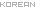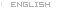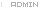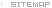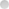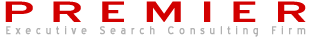<% code = "notice" sql = "select top 4 * from cs_bbs_data where code='"& code &"' order by idx desc" set rs = dbcon.execute(sql) do while not rs.eof subject = left(rs("subject"),30) %> <% rs.movenext loop %>"><%=subject%>프리미어의 홈페이지를 새롭게 리뉴얼 하였습니다.프리미어의 홈페이지를 새롭게 리뉴얼 하였습니다.프리미어의 홈페이지를 새롭게 리뉴얼 하였습니다.프리미어의 홈페이지를 새롭게 리뉴얼 하였습니다.
<% '팝업창 로직 syear = Year(date) smonth = Month(date) if len(smonth)=1 then smonth = "0"&smonth end if sday = Day(date) if len(sday)=1 then sday = "0"&sday end if odate = syear&smonth&sday sql = "select * from cs_popup order by idx asc" set rs = dbcon.execute(sql) do while not rs.eof if request.cookies("popup_cookie_"&rs("idx")) <> "NO" then if rs("start_day")<=odate and rs("end_day")>=odate then heights = rs("height") + 24 %> <% end if end if rs.movenext loop '팝업창 로직 완료 %>